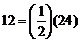# Introduction

## Factoring polynomials

Factoring is the process by which we go about determining what we multiplied to get the given quantity. An important part of this process is being able to express a complicated polynomial into a product of simpler polynomials. This involves factorisation.

We do this all the time with numbers. For instance, here are a variety of ways to

factor 12.

 12 = (2) (6) 12 = (3) (4) 12 = (2) (2) (3)12 = (–2) (–6) 12 = (–2) (2) (–3)

There are many more possible ways to factor 12, but these are representative of many of them. A common method of factoring numbers is to completely factor the number into positive prime factors. A prime number is a number whose only positive factors are 1 and itself. For example 2, 3, 5, and 7 are all examples of prime numbers. Examples of numbers that aren’t prime are 4, 6, and 12 to pick a few.

If we completely factor a number into positive prime factors there will only be one way of doing it. That is the reason for factoring things in this way. For our example above with 12 the complete factorization is,

 12 = (2) (2) (3) 12 = (2) (2) (3)

Here are a couple of examples.

x2 – 16 = (x + 4) (x – 4)

This is completely factored since neither of the two factors on the right can be further factored.

Likewise,

x4 – 16 = (x2 + 4) (x2 – 4)

is not completely factored because the second factor can be further factored. Note that the first factor is completely factored however. Here is the complete factorization of this polynomial.

x4 – 16 = (x2 + 4) (x + 2) (x – 2)

The purpose of this section is to familiarize ourselves with many of the techniques for factoring polynomials.

To score More in your class 7 refer NCERT solutions for class 7 Maths